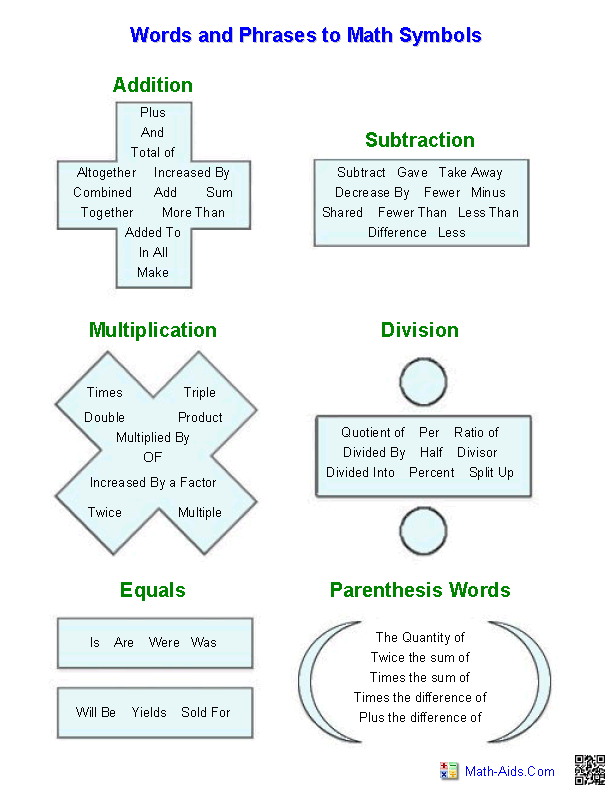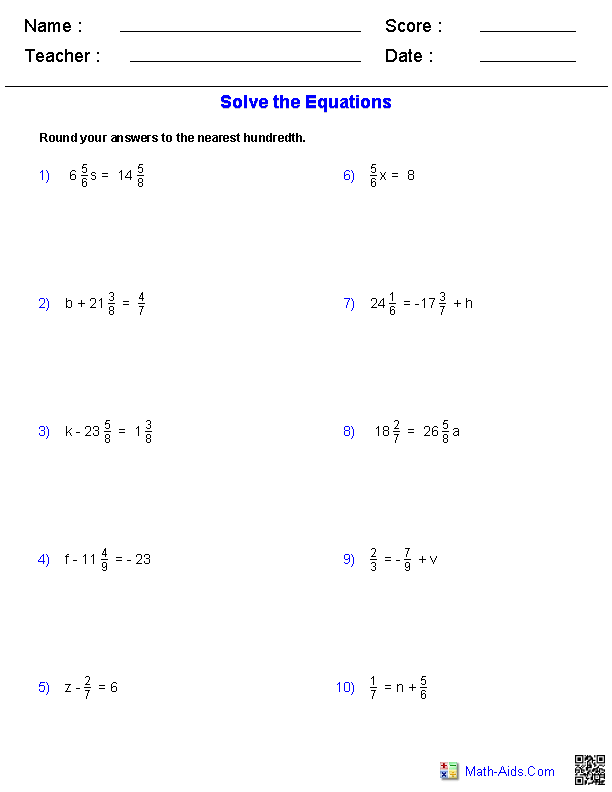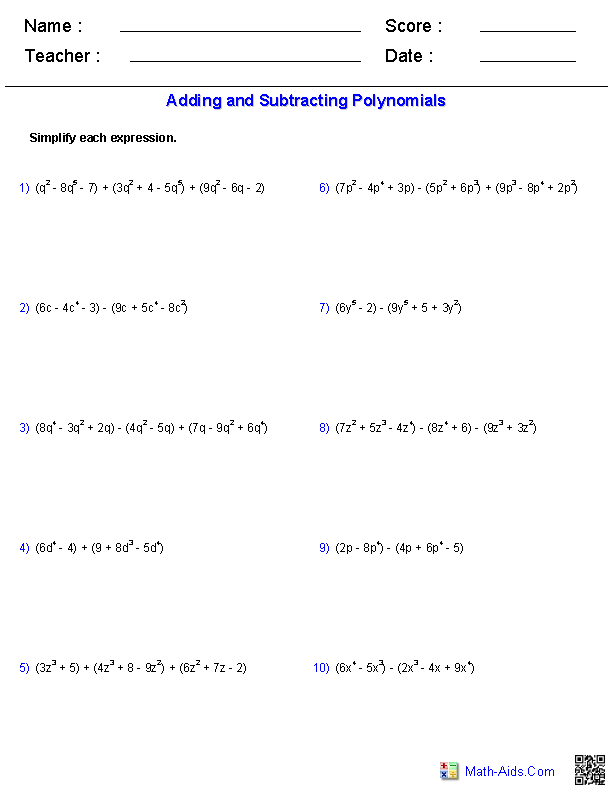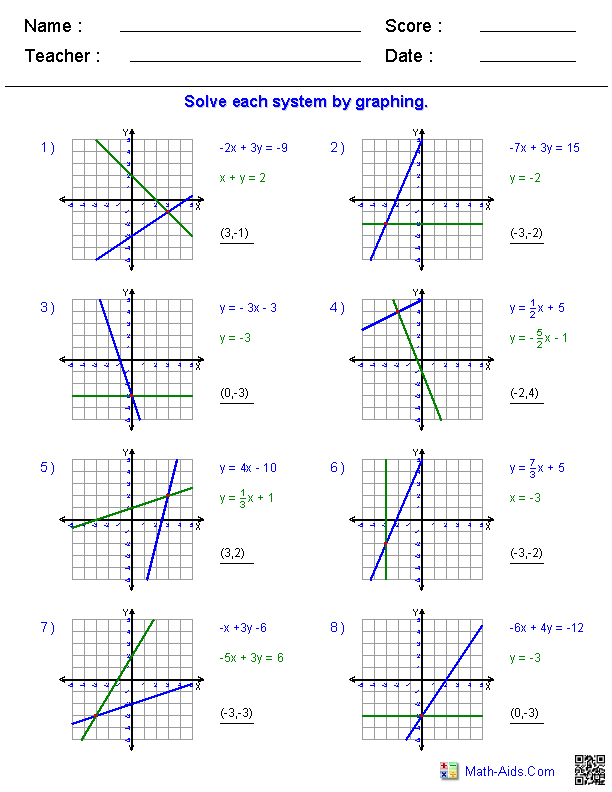#Pre-Algebra Worksheets

## Dynamically Created Pre-Algebra Worksheets

Here is a graphic preview for all of the Pre-Algebra Worksheet Sections. You can select different variables to customize these Pre-Algebra Worksheets for your needs. The Pre-Algebra Worksheets are randomly created and will never repeat so you have an endless supply of quality Pre-Algebra Worksheets to use in the classroom or at home. We have six different topics covering Algebraic Expressions, Inequalities, Equations, Linear Functions, Polynomials, and Systems of Equations.

Our Pre-Algebra Worksheets are free to download, easy to use, and very flexible.

These Pre-Algebra Worksheets are a good resource for students in the 5th Grade through the 8th Grade.

Click here for a Detailed Description of all the Pre-Algebra Worksheet Sections.

## Quick Link for All Pre-Algebra Worksheet Sections

Click the image to be taken to that Pre-Algebra Worksheet Section.

##### Algebraic ExpressionsPre-Algebra Worksheets##### Equations WorksheetsPre-Algebra Worksheets##### Inequalities WorksheetsPre-Algebra Worksheets##### Linear Functions WorksheetsPre-Algebra Worksheets##### Monomials and PolynomialsPre-Algebra Worksheets##### Systems of EquationsPre-Algebra WorksheetsRecommended Videos

## Detailed Description for All Pre-Algebra Worksheet Sections

Algebraic Expressions Worksheets
This section contains all of the graphic previews for the Algebraic Expressions Worksheets. We currently have topics covering Translate Phrases, Simplifying Variable Expressions, Evaluating Variable Expressions, Simplifying Variable Expressions Using the Distributive Property, Evaluating One Variable Expressions, and Evaluating Two Variable Expressions. These Algebraic Expressions Worksheets are a good resource for students in the 5th Grade through the 8th Grade.

Equations Worksheets
This section contains all of the graphic previews for the Equations Worksheets. We currently have topics covering Modeling Equations, One-Step Equations, Two-Step Equations, and Multi-Step Equations. These Equations Worksheets are a good resource for students in the 5th Grade through the 8th Grade.

Inequalities Worksheets
This section contains all of the graphic previews for the Inequalities Worksheets. We currently have topics covering Graphing Single-Variable Inequalities, One-Step Inequalities by Adding and Subtracting, One-Step Inequalities by Multiplying or Dividing, Two-Step Inequalities, and Multi-Step Inequalities. These Inequalities Worksheets are a good resource for students in the 5th Grade through the 8th Grade.

Linear Functions Worksheets
This section contains all of the graphic previews for the Linear Functions Worksheets. We currently have topics covering Finding Slope, Graphing Lines Using Slope-Intercept Form, Graphing Lines Using Standard Form, Writing Linear Equations, and Graphing Linear Inequalities. These Linear Functions Worksheets are a good resource for students in the 5th Grade through the 8th Grade.

Monomials and Polynomials Worksheets
This section contains all of the graphic previews for the Monomials and Polynomials Worksheets. We currently have topics covering Factoring Monomials, Adding and Subtracting Polynomials, Multiplying a Monomial and a Polynomial, and Multiplying Binomials. These Monomials and Polynomials Worksheets are a good resource for students in the 5th Grade through the 8th Grade.

Systems of Equations Worksheets
This section contains all of the graphic previews for the Systems of Equations Worksheets. We currently have topics covering Solving Systems of Equations by Graphing, Solving Systems of Equations by Substitution, and Systems of Equations Word Problems. These Algebra 2 worksheets are a good resource for students in the 5th Grade through the 8th Grade.